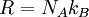# Molar gas constant

The molar gas constant,$R$, is given by$R = N_A k_B$